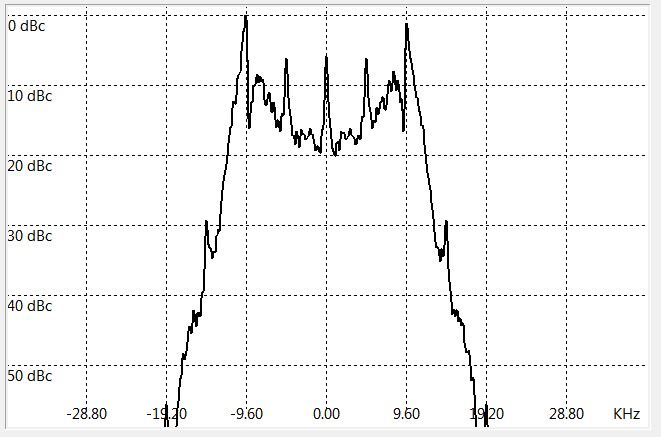If you have a related question, please click the "Ask a related question" button in the top right corner. The newly created question will be automatically linked to this question.

# CC1120: Carlson's Rule(s)

Part Number: CC1120
Other Parts Discussed in Thread: CC1121

Looking for clarity & finality on this subject...

I have seen (5) different iterations on Carlson's Rule for calculating transmitter bandwidth each producing (5) different results.

If dealing with a simple 2-level FSK signal and a simple bit rate, can the formula be simplified or reduced to:

Bandwidth = BPS + Separation

where BPS is 2 x the Modulation Frequency and Separation is 2 x the Frequency Deviation.

So, for a BPS of 4.8KHz and a Separation of 20K, the Bandwidth is 24.8KHz?

(The period of 4.8KBPS being actually 2.4KHz and the Separation being actually the total +/- offset from the CW center frequency.)

Part of the ambiguity that I have seen is Deviation is not always qualified as being strictly a (+/-) figure but sometimes is actually total Deviation (aka Separation).

Thanks,

--

Jay Zebryk

• Carson's rule (signal BW) = 2 x fm + 2 x deviation

fm is the highest modulating frequency and equals bit rate/2 for 2-(G)FSK (0-1 sequence)
deviation is separation / 2

Thus, the formula can be simplified to signal BW = bit rate + separation

Note that if you use Carson's rule to estimate the required RX filter BW (> signal bandwidth) you also need to take into account the frequency offset between the RX and TX units.

• Sverre,

So, for a BPS of 4.8KHz and a Separation of 20K, the Bandwidth is 24.8KHz. Correct?

Quite vital that I get this right as the figure feeds into my crystal tolerance requirement calculations.

Thanks,
• Using Carson's rule the signal BW = bit rate + separation

bit rate = 4.8 kbps, separation = 20 kHz

signal BW = 4.8 + 20 = 24.8 kHz

Using a more accurate simulation model the 99% occupied bandwidth is 23.4 kHz when using GFSK modulation (FSK = 31.6 kHz). Plot below is GFSK modulation.• Yikes!

So, does this mean Carlson's Rule is void?

Experimenting with a RF Generator and a Spectrum Analyzer, this appears to be so.

(See below; CC1121 set to +/-10KHz (20KHz Separation) @4800BPS

Of the various combinations of Carlson, 2 x BPS + Separation = 29.6KHz is much closer to 31.6KHz

Zebryk's Rule of Thumb:-)

How do we resolve this?

For my immediate project, how confident are you in your Mathlab figure of 31.6KHz?

(Please recall this is playing against the CC1121's RX Bandwidth set to 41.667KHz which is the minimum for that part.)

--

Jay Zebryk

• Carson rule is (fairly) valid for a perfect FSK signal. GFSK uses shaping on the symbols meaning that a GFSK spectrum will be narrower than a FSK spectrum. So if you use GFSK carsons rule will give you a too large value. I mainly use Carsons rule as a "rule of thumb" and then use a Matlab script to give a more accurate number.

Do you send random GFSK for your plot? It looks more like preamble and FSK.
• TER,

This pertains only to FSK.
The output seen was generated using a TrxEB with a CC1121 EVM set for continuous TX with "Random Modulation" mode in SmartRF Studio.

For the purposes of determining the RX bandwidth required for a certain OBW, it seems Carlson's rule not only obsolete but only rough at best and should no longer be promoted.

So, this NOT being a theoretical exercise, I need to determine the maximum OBW for the CC1121 RX bandwidth set to 41.667KHz.

Once that can be firmly established, I then can feed that into your Crystal Tolerance spreadsheet tool, to figure what PPM is required for both TX and RX.

The uncertainty of this spawns more questions such as:

1) What is the tolerance of a 41.667KHz RXbw in terms of maximum TXbw?
2) What % Power should TXbw be used . (95%-99%)
3) What additional normal part-to-part tolerance(s) should be included into the above calculations?

Thanks,

--
Jay Zebryk
• The Matlab plot is calculated from an ideal FSK. In the real world the time domain of FSK will not have an instantaneous transition from -dev to +dev, some small shaping of the symbols will be the case. Therefore a real world spectrum would most likely be narrower than the Matlab calculation.

In the spectrum plot you posted, what percent does you spectrum use as OBW?

1) Not quite sure if I understand the question. The Rx Bandwidth is set by a brick wall digital filter and the Rx bandwidth will therefore only have a small variation due to the xtal accuracy.
2) I tend to use 95 %
3) The Tx bandwidth is set digitally. Hence the part to part tolerance will only be set by the xtal accuracy.
• TER,

Understood about the difference between Matlab and reality.
My rushed measurement before breakfast was set at 99%.
I will repeat it @95%.

Still, I seek direction how best to resolve this properly.

Is there no better formula available than Carlson's?
Is this something which requires RF measurement on a case to case basis for all future projects?

Before we toss Carlson, I have seen many various versions of his formula one of which is on Wikepedia:

en.wikipedia.org/.../Carson_bandwidth_rule

Note this is expressed as 2 x (ModHz + Deviation)
2 x (4.8KHz + 10K) = 29.6KHz which is much closure to the 95% measured bandwidth.
4.8KHz being the highest frequency input; nothing to do with the 2.4KHz modulation frequency.
10KHz being (+/-) Deviation; not 20KHz which is the Separation.

This, although apparently "more" valid, my confidence in that interpretation is not 100%, even as a Rule of Thumb.